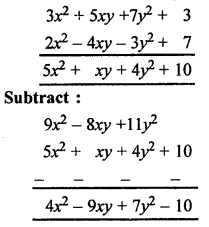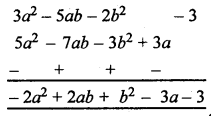## ML Aggarwal Class 8 Solutions for ICSE Maths Chapter 10 Algebraic Expressions and Identities Ex 10.1

Question 1.
Identify the terms, their numerical as well as literal coefficients in each of the following expressions:
(i) 12x2yz – 4xy2
(ii) 8 + mn + nl – lm
Solution:Question 2.
Identify monomials, binomials, and trinomials from the following algebraic expressions :
(i) 5p × q × r2
(ii) 3x2 + y ÷ 2z
(iii) -3 + 7x2
(iv) $$\frac{5 a^{2}-3 b^{2}+c}{2}$$
(v) 7x5 – $$\frac{3 x}{y}$$
(vi) 5p ÷ 3q – 3p2 × q2
Solution:
(i) 5p × q × r2 = 5pqr2
In this algebraic expression having only one term
therefore it is monomial.

(ii) 3x2 + y ÷ 2z = $$\frac{3 x^{2} y}{2 z}$$
In this algebraic expression having only one term
therefore it is monomial.

(iii) -3 + 7x2
In this algebraic expression having two terms
therefore it is binomial.

(iv) $$\frac{5 a^{2}-3 b^{2}+c}{2}=\frac{5 a^{2}}{2}-\frac{3 b^{2}}{2}+\frac{c}{2}$$
In this algebraic expression having three terms
therefore it is trinomial.

(v) 7x5 – $$\frac{3 x}{y}$$
In this algebraic expression having two terms
therefore it is binomial.

(vi) 5p ÷ 3q – 3p2 × q2 = $$\frac{5 p}{3 q}$$ = 3p2q2
In this algebraic expression having two terms
therefore it is binomial.

Question 3.
Identify which of the following expressions are polynomials. If so, write their degrees.
(i) $$\frac{2}{5}$$x4 – $$\sqrt{3}$$x2 + 5x – 1
(ii) 7x3 – $$\frac{3}{x^{2}}$$ + $$\sqrt{5}$$
(iii) 4a3b2 – 3ab4 + 5ab + $$\frac{2}{3}$$
(iv) 2x2y – $$\frac{3}{x y}$$ + 5y3 + $$\sqrt{3}$$
Solution:
(i) Polynomial, and degree of this expression is 4.
(ii) Not a polynomial.
(iii) Polynomial, and degree of this expression is 5.
(iv) Not a polynomial.

Question 4.
(i) ab – bv, bv – ca, ca – ab
(ii) 5p2q2 + 4pq + 7,3 + 9pq – 2p2q
(iii) l2 + m2 + n2, lm + mn, mn + nl, nl + lm
(iv) 4x3 – 7x2 + 9, 3x2 – 5x + 4, 7x3 – 11x + 1, 6x2 – 13x
Solution:
(i) ab – bc, bc – ca, ca – ab
= ab – bc + bc – ca + ca – ab = 0

(ii) 5p2q2 + 4pq + 7,3 + 9pq – 2p2q2
= 5p2q2 + 4pq + 7 + 3 + 9pq – 2p2q2
= 5p2q2 – 2p2q2 + 4pq + 9pq + 7 + 3
= 3p2q2 + 13pq + 10

(iii) l2 + m2 + n2, lm + mn, mn + nl, nl + lm
= l2 + m2 + n2 + lm + mn + mn + nl + nl + lm
= l2 + m2 + n2 + 2lm + 2mn + 2nl

(iv) 4x3 – 7x2 + 9, 3x2 – 5x + 4, 7x3 – 11x + 1, 6x2 – 13x
= 4x3 – 7x2 + 9 + 3x2 – 5x + 4 + 7x3 – 112 + 1 + 6x2 – 13x
= 4x2 + 7x3 – 7x2 + 3x2 + 6x2 – 5x – 11x – 13x + 9 + 4 + 1
= 11x3 – 2x2 – 29x + 14

Question 5.
Subtract:
(i) 8a + 3ab – 2b + 7 from 14a – 5ab + 7b – 5
(ii) 8xy + 4yz + 5zx from 12xy – 3yz – 4zx + 5xyz
(iii) 4p2q – 3pq + 5pq2 – 8p + 7q -10 from 18 – 3p – 11q + 5pq – 2pq2 + 5p2q
Solution:
(i) 8a + 3ab – 2b+ 1 from 14a – 5ab + 7b – 5
= (14a – 5ab + 7b – 5) – (8a + 3ab – 2b + 7)
= 14a – 5ab + 7b – 5 – 8a – 3ab + 2b – 7
= 6a – 8ab + 9ab – 12

(ii) 8xy + 4yz + 5zx from 12xy – 3yz – 4zx + 5xyz
= (12xy – 3yz – 4zx + 5xyz) – (8xy + 4yz + 5zx)
= 12xy – 3yz – 4zx + 5xyz – 8xy – 4yz – 5zx
= 4xy – 7yz – 9zx + 5xyz

(iii) 4p2q – 3pq + 5pq2 – 8p + 7q – 10
from 18 – 3p – 11q + 5pq – 2pq2 + 5p2q
= (18 – 3p – 11q + 5pq – 2pq2 + 5p2q) – (4p2q – 3pq + 5pq2 – 8p + 7q – 10)
= 18 – 3p – 11q + 5pq – 2pq2 + 5p2q – 7p2q + 3pq – 5pq2 + 8p – 7q + 10
= 28 + 5p – 78q + 8pq – 7pq2 + p2q

Question 6.
Subtract the sum of 3x2 + 5xy + 7y2 + 3 and 2x2 – 4xy – 3y2 + 7 from 9x2 – 8xy + 11y2
Solution:
Sum :Question 7.
What must be subtracted from 3a2 – 5ab – 2b2 – 3 to get 5a2 – 7ab – 3b2 + 3a ?
Solution:
We have to subtract 5a2 – 7ab – 3b2 + 3a
from 3a2 – 5ab – 2b2 – 3Question 8.
The perimeter of a triangle is 7p2 – 5p + 11 and two of its sides are p2 + 2p – 1 and 3p2 – 6p + 3. Find the third side of the triangle.
Solution:
We know that,
Perimeter of a triangle = Sum of three sides of triangle
⇒ 7p2 – 5p + 11 = (p2 + 2p – 1) + (3p2 – 6p + 3) + (Third side of triangle)
⇒ 7p2 – 5p + 11 = (4p2 – 4p + 2) + (Third side of triangle)
⇒ Third side of triangle
= (7p2 – 5p + 11) – (4p2 – 4p + 2)
= (7p2 – 4p2) + (- 5p + 4p) + (11 – 2)
= 3p2 – p + 9
Hence third side of triangle is 3p2 – p + 9